﻿ Validation of Results Obtained from Different Types of Fuzzy Controllers for Diagnosis of Inclined Edge Crack in Cantilever Beam by Vibration Parameters

### Validation of Results Obtained from Different Types of Fuzzy Controllers for Diagnosis of Inclined E...

Ranjan K. Behera, Dayal R. ParhiOPEN ACCESSPEER-REVIEWED

## Validation of Results Obtained from Different Types of Fuzzy Controllers for Diagnosis of Inclined Edge Crack in Cantilever Beam by Vibration Parameters

Ranjan K. Behera1,, Dayal R. Parhi1

1Department of Mechanical Engineering, National Institute of Technology, Rourkela, Odisha, India

### Abstract

In this paper, the crack diagnosis using intelligent techniques (using membership functions in different fuzzy controllers) have been developed for inverse investigation of the vibration parameters (like modal frequencies and mode shapes) and crack parameters (like crack location, crack depth and crack inclination) of an inclined edge crack cantilever beam. The vibration parameters are calculated from finite element (using ANSYS) and experimental analysis which are used as inputs to the different fuzzy controllers. The different fuzzy controllers are designed by taking several types of membership functions to calculate the crack parameters. The calculated first three modal frequencies and mode shapes are used to generate the number of fuzzy rules with three output crack parameters. Finally, the proposed intelligent techniques are validated by comparing the results obtained from both FEA and experimental analysis. All the results are obtained from fuzzy controllers are in good agreement with experimental results.

### At a glance: Figures

12345
Prev Next

• Behera, Ranjan K., and Dayal R. Parhi. "Validation of Results Obtained from Different Types of Fuzzy Controllers for Diagnosis of Inclined Edge Crack in Cantilever Beam by Vibration Parameters." Journal of Mechanical Design and Vibration 2.3 (2014): 63-68.
• Behera, R. K. , & Parhi, D. R. (2014). Validation of Results Obtained from Different Types of Fuzzy Controllers for Diagnosis of Inclined Edge Crack in Cantilever Beam by Vibration Parameters. Journal of Mechanical Design and Vibration, 2(3), 63-68.
• Behera, Ranjan K., and Dayal R. Parhi. "Validation of Results Obtained from Different Types of Fuzzy Controllers for Diagnosis of Inclined Edge Crack in Cantilever Beam by Vibration Parameters." Journal of Mechanical Design and Vibration 2, no. 3 (2014): 63-68.

 Import into BibTeX Import into EndNote Import into RefMan Import into RefWorks

### 1. Nomenclature

L: Length of the cantilever beam

b: Width of the cantilever beam

t: Thickness of the cantilever beam

a: Edge Crack depth

L1: Crack location from fixed end of the cantilever beam

β: Relative crack location

α: Relative crack depth

θ: Crack inclination angle

RFNF : Relative First Natural Frequency

RSNF : Relative Second Natural Frequency

RTNF: Relative Third Natural Frequency

RFMD: Relative First Mode Difference

RSMD: Relative Second Mode Difference

RTMD: Relative Third Mode Difference

RCL: Relative Crack Location

RCD: Relative Crack Depth

CA: Crack Angle

### 2. Introduction

Early identification of cracks in engineering dynamic structure like beams during their life time is the main task to the researchers. Though vibration based crack analysis has been advanced for last three decades and, still there are various difficulties occur during application. There are various methods to calculate the problem of a cracked beam such as theoretical, analytical, experimental etc. FEM (Finite Element Method) is a general method to get the stiffness matrix of the cracked beam element. Many researchers are using vibration parameters like stiffness, natural frequencies and mode shapes to determine the position and depth of the crack in the beam. The natural frequencies decrease due to the cracks in the beam because of decrease the stiffness.

Shih et al.  determined fault identification in structures like beams and plates by using multi-criteria approach which includes two methods, one is modal flexibility and another is modal strain energy method. After getting results from above two methods are used in algorithms for identify single and multiple damages having different boundary conditions. Prasad et al.  investigated that the effect of crack position from free end to fixed end of the vibrating cantilever beam at each of the frequency on the resolve of crack growth rate. Mazanoglu and Sabuncu  have presented an algorithm for crack detection from searching over the frequency map and minimizing the measurement errors. Also a statistical approach called ‘recursively scaled zoomed frequencies (RSZF)’ is used for reducing the deviations. B. P. Nandwana and S. K. Maiti  have determined the crack position, crack depth and crack inclination by using finite element method and experimental method. The inclined crack used as a rotational spring for vibrational analysis which helps to determine the crack location and depth, according to the changes of vibration signatures. Kalanad and Rao  have measured relative crack depth up to 0.9 times of total element depth and natural frequency of cracked beam more accurately using two dimensional finite element approaches. The function of frequency response and function of crack size and location are approached by means of surface-fitting techniques. Bing et al.  identified the multiple crack of beam by using a three­step­meshing method with less subdivision and more precision. It can be used to detection of multiple cracks of complicated structure. Kim et al.  described a computer based crack analysis system for concrete structures using Fuzzy logic theory. When they used this theory the approach to identify the crack the planned system delivered results like to those got by experts system. Saravanan et al.  proposed a method based on the vibration signals developed from the operating machines to effectively detect the conditions of unreachable moving components inside the machine. The proposed model was designed using fuzzy classifier to generate the rules automatically from the feature set. Boutros and Liang  established four condition monitoring indicators for identification of transient and gradual abnormalities using fuzzy logic method. They successfully examined and validated the fuzzy-based method in two different applications. Sugumaran and Ramachandran  described the use of decision tree of a fuzzy classifier for choosing top few features that will distinguish the fault condition of the bearing from given trained samples. Miguel and Blazquez  established a decision making module based on fuzzy logic for model based damage analysis applications.

The objective of this paper, intelligent techniques have been developed using different types of membership functions (MF) in fuzzy controllers for crack prognosis in a cantilever beam having inclined crack. The first three natural frequencies of the cracked cantilever beam structure have been calculated from both FEA (using commercial available ANSYS 12  software) and experimental analysis. The results obtained from both the analysis have been presented. An inverse analysis has been carried out using fuzzy controllers for inclined crack identification. The results obtained from experimental analysis have been compared with the results obtained from both fuzzy controllers and FEA and a good agreement have been observed between the results.

### 3. Design Limitations

The continuous model of the beam has been discretized for simplification. According to B. P. Nandwana and S. K. Maiti , for inclined edge crack cantilever beams,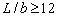,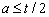and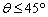, the difference in the two extreme locations is less than 4% of the beam length. The relative crack position range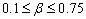from fixed end and relative edged crack depth rangeare tested.

### 4. Vibration Analysis of Inclined Crack Cantilever Beam

The beam with an inclined edge crack is fixed at left end, free at right end and it has a uniform structure having constant rectangular cross-section. The Euler–Bernoulli beam model was assumed. The crack is considered as an open crack and the damping has ignored in this study. The free bending vibration of an Euler-Bernoulli beam of a constant rectangular cross section (Figure 1 and Figure 2) is given by the following governing equation: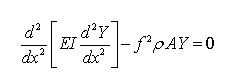(1)

Whereis displacement,is the cross-sectional area,is the mass density,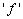is natural frequency of the vibration of the beam,is the moment of inertia,is the Young's modulus of elasticity and the x-axis is aligned with the axis of the beam. The equations for the each part are given below: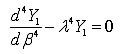(2)(3)

Hereandare non-dimensional parameters. Solving eqs (2) and (3), we get displacements on each part of the beam(4)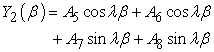(5)

where A1 to A8 are arbitrary constants to be calculated from the boundary conditions. The boundary conditions at the fixed and free ends, respectively, are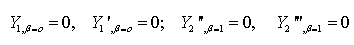(6)

The continuity of displacement, bending moment and shear forces at the crack location (say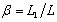) and jump condition in the slope can be written in the following form: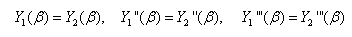(7)

### 5. Finite Element Analysis (FEA)

The finite element analysis is carried out using ANSYS 12 finite element program for modal analysis of the un-cracked and inclined edge crack cantilever beam to determine the natural frequencies at different crack location, crack depth and crack inclination. Figure 3 shows the finite element mesh model of the cantilever beam and Figure 4 shows a magnified view of three dimensional inclined open edge crack zone.

The cracked beams of the present analysis have the following configurations.

Length of the Beam (L)=800mm

Width of the beam (b)=60mm

Thickness of the Beam (t)=6mm

Relative crack depth (α)=Varies from 0.1 to 0.5Relative crack location (β) =Varies from 0.1 to 0.75

The material properties are taken for analysis as given below.

Young's modulus of elasticity (E)=70GPa

Poisson’s ratio (ν) =0.346

Density (ρ) =2710Kg/m3

The first three natural frequencies and mode shapes are calculated by FEA.

### 6. Experimental Analysis

The experiment on cantilever beam has been done for verify the FEA and fuzzy logic results. The aluminum cantilever beam specimen with dimensions (800mm x 60mm x 6 mm) with and without a crack is subjected to number of experimentation with the experimental set up shown in Figure 6 and Figure 5 for determining the natural. An experimental set-up contains (1) Vibration Analyzer, (2) Accelerometer, (3) Vibration Indicator, (4) Vibration Exciter, (5) Power Amplifier, (6) Test Specimen-beam, (7) Power Distribution and (8) Function Generator are used for performing the experiment as shown Figure 5 in the schematic diagram.

6.1. Specifications of the Instruments Used in the Experimental Set up

(1) Vibration Analyzer

Type: 3560L

Make: Bruel & kjaer

Frequency: 7 Hz to 20 Khz

Channels: 2 Inputs, 2 Tachometer

Input Type: Direct/CCLD

(2) Accelerometer

Type: 4513-001

Make: Bruel & kjaer

Sensitivity: 10mv/g-500mv/g

Frequency Range: 1Hz-10KHz

Supply voltage: 24volts

(3) Vibration Indicator

Software: PULSE LabShop Software Version 12

Make: Bruel & kjaer

(4) Vibration Exciter

Type: 4808

Force rating: 112N (25 lbf) sine peak )

Frequency: 5Hz to 10 kHz

First axial resonance: 10 kHz

(5) Power Amplifier

Type: 2719

Power Amplifier: 180VA

Make: Bruel & kjaer

(6) Test Specimen-beam

Material: Aluminum

Dimension: 800mmX60mmX6mm

(7) Power Distribution

220V power supply, 50Hz

(8) Function Generator

Model: FG200K

Frequency Range: 0.2Hz to 200 KHz

Output Level: 15Vp-p into 600 ohms

Before the experimental study the beams surface has been cleaned and organized for straightness. Subsequently, transverse inclined crack is created at different location from fixed end in different specimens with the help of Wire EDM machine. The natural frequencies corresponding to1st, 2nd and 3rd mode are noted with different crack depth at different crack locations and different crack inclinations in the cracked cantilever beam.

6.2. Experimental Results

The mode shapes obtained from experimentation (Figure 5) for different relative crack locations, relative crack depths and crack angles have been compared with that of the finite element analysis (FEA) for both cracked and uncracked beam. The comparison results are shown in Figure 6 to Figure 11.

Figure 7. Magnified view of First mode relative amplitude Vs. Relative location from cantilever end at β = 0.3, α = 0.35 and Ɵ = 35°
Figure 9. Magnified view of Second mode relative amplitude Vs. Relative location from cantilever end at β = 0.3, α = 0.35 and Ɵ = 35°
Figure 11. Magnified view of Third mode relative amplitude Vs. Relative location from cantilever end at β = 0.3, α = 0.35 and Ɵ = 35°

### 7. Analysis of the Fuzzy Controller

The different fuzzy controllers have developed for analysis of crack detection. Here only one fuzzy controller (Gaussian Fuzzy Controller) having six input and three output parameters as shown in Figure 12. The linguistic term used for the inputs are as follows; “RFNF”; “RSNF”, “RTNF”, “RFMD”, “RSMD” & “RTMD” and the linguistic term used for the outputs are as follows; “RCL”; “RCD” & “CA”. For each fuzzy controller there nine MFs have taken for each six input parameters (“RFNF”, “RSNF”, “RTNF”, “RFMD”, “RSMD” & “RTMD”) and nineteen MFs for “RCL”, nine MFs for “RCD” and twenty nine MFs for “CA” have taken as shown in Figure 13, Figure 14 & Figure 15. More than one hundred fuzzy rules have developed and have found the output results close to the experimental results. The crisp values of RCL, RCD and CA are computed using the center of gravity method as given below.#### Table 1. Comparison between FEA, Gaussian, Trapezoidal and Bell Shape Fuzzy Controllers with Experimental results of inclined edge crack in cantilever beamsDownload asPowerPoint Slide

Veiw figureView current table in a new window

### 8. Discussion

The results found from finite element, fuzzy and experimental analysis is discussed in the following section. The each fuzzy controller is designed with fuzzy Gaussian, Trapezoidal and Bell Shape membership functions. An experimental set-up (as shown in Figure 5) has been developed to validate the results from the various methodologies adopted in the current analysis by performing experiments on an Aluminum beam specimen. The results from FEA, different fuzzy controller and experimental analysis are compared as shown in Table 1. Thus, the intelligent fuzzy controller can be successfully used for structural health monitoring.

Figure 15. Gaussian MF for Relative Crack Location, Relative Crack Depth and Crack Angle vs. Degree of Membership Respectively

### 9. Conclusion

The conclusions derived from the various analyses as mentioned above are depicted below.

•  The results from finite element analysis are good agreement with the results obtained from fuzzy logic analysis.

•  The analysis of vibration characteristics of the cracked beam shows a variation of mode shapes and natural frequencies for the cracked and un-cracked beam.

•  The results obtained from finite element analysis, fuzzy analysis are compared with the experimental results. They show a very good agreement.

•  This technique can be used as a condition monitoring tool for vibrating fault structures like beams and plates.

### References

  H.W. Shih, D.P. Thambiratnam and T.H.T. Chan, “Vibration based structural damage detection in flexural members using multi-criteria approach,” Journal of Sound and Vibration, vol. 323, pp. 645–661, 2009.J. Clerk Maxwell, A Treatise on Electricity and Magnetism, 3rd ed., vol. 2. Oxford: Clarendon, 1892, pp.68-73.In article  RS Prasad, SC Roy and KP Tyagi, “Effect of Crack Position along Vibrating Cantilever Beam on Crack Growth Rate,” International Journal of Engineering Science and Technology, Vol. 2(5), pp. 837-839, 2010.In article  K. Mazanoglu and M. Sabuncu, “A frequency based algorithm for identification of single and double cracked beams via a statistical approach used in experiment,” Mechanical Systems and Signal Processing, vol. 30, pp. 168-185, 2012.In article CrossRef  B. P. NANDWANA and S. K. MAITI, “Modelling Of Vibration Of Beam In Presence Of Inclined Edge Or Internal Crack For Its Possible Detection Based On Frequency Measurements,” Engineering Fracture Mechanics, Vol. 58, No. 3, pp. 193-205, 1997.In article CrossRef  Aysha Kalanad and B. N. Rao, “Detection of crack location and size in structures using improved damaged finite element,” IOP Conf. Series: Materials Science and Engineering, vol. 10, pp. 012054, 2010.In article  LI Bing, CHEN Xuefeng, and HE Zhengjia, “Three­Steps­Meshing based Multiple Crack Identification for Structures and Its Experimental Studies,” Chinese journal of mechanical engineering, vol. 26, no. 1, pp. 1-7, 2013.In article  Y. M. Kim, C. K. Kim, and G. H. Hong, “Fuzzy set based crack diagnosis system for reinforced concrete structures,”Comput-ers and Structures, vol. 85, no. 23-24, pp. 1828-1844, 2007.In article CrossRef  N. Saravanan, V. N. S. K. Siddabattuni, and K. I. Ramachandran, “Fault diagnosis of spur bevel gear box using artificial neural network (ANN), and proximal support vector machine (PSVM),”Applied Soft Computing Journal,vol.10, no.1, pp. 344-360, 2010.In article CrossRef  T. Boutros and M. Liang, “Mechanical fault detection using fuzzy index fusion,”International Journal of Machine Tools and Manufacture, vol. 47, no. 11, pp. 1702-1714, 2007.In article CrossRef  V. Sugumaran and K. I. Ramachandran, “Fault diagnosis of roller bearing using fuzzy classifier and histogram features with focus on automatic rule learning,”Expert Systems with Applications, vol. 38, no. 5, pp. 4901-4907, 2011.In article CrossRef  L.J.De Miguel and L.F.Bl´ azquez, “Fuzzy logic-based deci-sion-making for fault diagnosis in a DC motor,”Engineering Applications of Artificial Intelligence, vol. 18, no. 4, pp. 423-450, 2005.In article CrossRef  ANSYS Release 12, Inc. engineering simulation software.In article